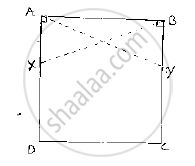Share
Notifications

View all notifications
Books Shortlist
Your shortlist is empty

# Abcd is a Square, X and Yare Points on Sides Ad and Bc Respectively Such that Ay = Bx. Prove that by = Ax and ∠Bay = ∠4bx. - CBSE Class 9 - Mathematics

Login
Create free account

Forgot password?
ConceptCriteria for Congruence of Triangles

#### Question

ABCD is a square, X and Yare points on sides AD and BC respectively such that AY = BX. Prove that BY = AX and ∠BAY = ∠ABX.

#### Solution

Given that ABCD is a square, X and Y are points on sides AD and BC respectively such that AY = BX.

We have to prove BY = AX and ∠BAY = ∠ABX
Join B and X, A and Y.

Since, ABCD is a square ⇒ ∠ DAB =  ∠CBA =90°

⇒  ∠XAB= ∠YBA=90°         .............(1)

Now, consider triangle XAB and YBA

We have

∠XAB=∠YBA=90°        ...........[From (1)]

BX=AY                                    [given]

And AB=BA                        [Common side]

So, by RHS congruence criterion, we have ΔXAB≅ΔYBA

Now, we know that corresponding parts of congruent triangles are equal.

∴ BY=AX and ∠BAY=∠ABX

∴ Hence provedIs there an error in this question or solution?

#### APPEARS IN

Solution Abcd is a Square, X and Yare Points on Sides Ad and Bc Respectively Such that Ay = Bx. Prove that by = Ax and ∠Bay = ∠4bx. Concept: Criteria for Congruence of Triangles.
S